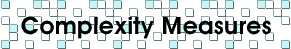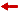### Lemoine's Measure

For every construction, Émile Lemoine defines its "simplicity" and its "exactitude" to be the total cost of the performed operations, according to the following table:

 operation simplicitycost exactitudecost To place a compass end on a given point. 1 1 To place a compass end on a given line. 1 1 To draw a circle when the compass is placed. 1 0 To place a ruler edge through a given point. 1 1 To draw a line when the ruler is placed. 1 0

On page 58 of , Coolidge notes:

"Lemoine's criteria are, perhaps, the simplest and easiest to apply; it is hard to imagine anything rougher."

He couldn't be more wrong: our complexity measure (number of lines + number of circles) is much simpler, easier and rougher!

Note that our measure can be given in terms of Lemoine's: it is simply the simplicity minus the exactitude.Back to The Complexity of Geometric Constructions